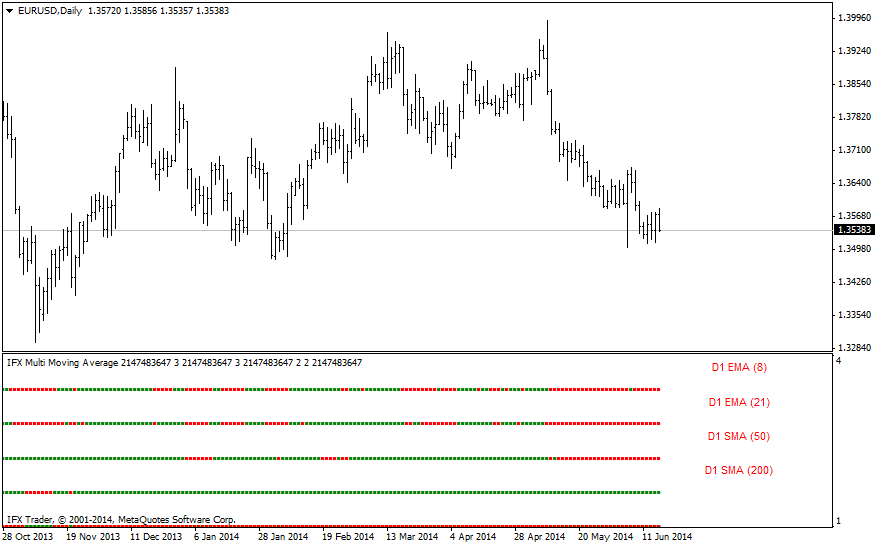#### Formula

SMA = Sum(Price)/n, where

Price — price at the moment from 1 to n;

n — moving average period.

#### Trading use

Various types of moving averages represent a popular analysis tool. Despite a large number of periods and types of MA calculation, practical use of moving averages is based on two factors: possibility to determine the trend direction with the help of MA and breakout/bounce trading from the level of this indicator.

Using IFX_MultiMovingAverage in MetaTrader, traders can easily figure out the trend showed by any of four moving averages applied in the indicator. The color of the indicator signals the trend direction: red color of moving averages in the extra window of the indicators means that the moving average is downward. Green color of IFX_MultiMovingAverage indicates an uptrend.

Using this indicator, traders can combine different moving averages, watching which trend prevails on a senior timeframe in one window. Based on this factor, traders can make market entry/exit decisions in the direction of the prevailing trend and at the same time monitor the moving average on a short-term time frame.#### IFX_MultiMA parameters

MA1 = 13

MA2 = 34

MA3 = 55

MA4 = 89

अभी बात नहीं कर सकते?
अपना प्रश्न पूछें बातचीत.